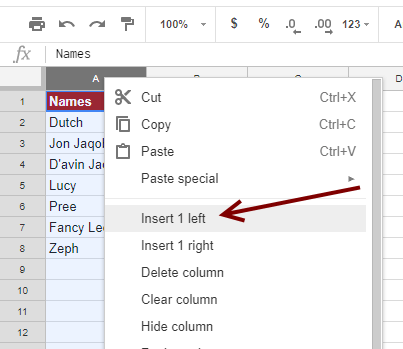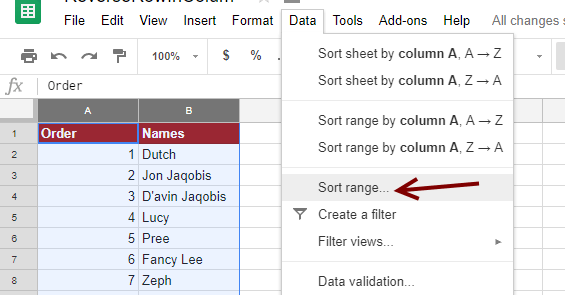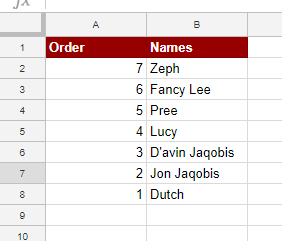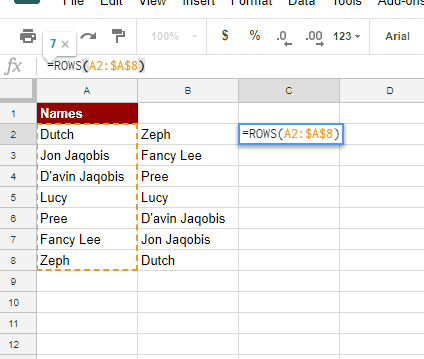# How do I reverse the Rows in A Column in Google Sheets?My favourite Killjoy members. Column A – From most favourite. Column B Saving the best for last.

Sometimes you have a need to reverse a list quickly in Google Sheets.

That’s pretty easy to do if the list is sorted alphabetically. Just go on into the <Data> menu and choose from one of the sort functions. But what if the data you want to flip is not in alphabetical or numeric order?

Below are 3 ways to reverse your data:

For the examples below, I’ll be using a list of my favourite Killjoys characters. Yeah, I’m a sci-fi geek.

##### Reverse Rows in a Column Using A Counter

top

This is probably the most formula-free way to flip a row in a column. This particular part of the tutorial is for the absolute beginner.

To achieve this task we are simply going to insert a column to the left of the Names column. Then number it from 1 to 7 and finally use that number to sort the names.

First, right-click on the top grey bar of Column A and then select <Insert 1 left>This will move the names to column B. Next, Name cell A1, “Order”. Then click cell A2 and type “1” and hit <Enter>. Click on cell A3 and type “2”. Don’t worry you don’t have to do it for all of them.

Click back on cell A2 and drag down to A3. You should see a blue border around the two cells with a little blue square on the right-hand bottom side. Click and drag that square down the page until you get to the end of your list.Finally, select columns A and B. Go to the <Data> menu. Then select <Sort range…>A pop-up window will appear. Tick the <Data has a header row> box. Sort by “Order” is fine unless you have your own location. Then select the Z-A radio button before clicking <Sort>The end result should look a little like this:If you don’t want the “Order” Column anymore you can simply right-click the column header and select <Delete Column>.

##### Reverse Rows in a Column Line By Line

top

To flip the Row’s in a Column in Google Sheets we can use the following formula:

`=INDEX(\$A\$2:\$A\$8,ROWS(A2:\$A\$8))`

This will go in the first cell. you will need to drag down the formula to the bottom of your list.

Let’s break it down.

We essentially want an INDEX of an item in each cell here. First, we need to select the range where we are going to find the data we want. You can see this with the first option: `=INDEX(\$A\$2:\$A\$8...` . Note the use of absolute reference as shown by “\$”.  When we drag this down to the bottom of the list we don’t want it to change.

Next, we use the ROWS function. ROWS gives us the number of rows in a selected range.  So we can assume that `ROWS(A2:\$A\$8))`  will return a value of 7. Note that we don’t set the first range value to absolute(`A2:`), only the last range value(`:\$A\$8`). This is because when we replicate the formula down the column we want the first value needs to change by one.

For example, the next cell down would be `ROWS(A3:\$A\$8)`. This would return a value of 6. Let’s take a look by just using the ROW function in column C.Now that we have those numbers we can use them in our INDEX function. The second parameter of the INDEX function is the row offset, or where in the range do we want to find our data. Because our data is 7 rows deep starting at A2 and our first offset, thanks to the first ROWS function, is 7 we know that the end result will be “Zeph” for the first entry.

The formula then might be explained like this:

`=INDEX(The range to find the data,Offset-ROWS(sum of rows between values))`

While useful for reversing one row in a column, it is not particularly helpful when you want to tie the other columns in the sheet to your flip altogether.

##### Reverse Rows in a Column With An Array

top

Finally, we can use the SORT function with the assistance of ROW. The SORT and ROW combination work in a similar manner to the INDEX and ROWS combination. However, SORT displays an array of information and that means a formula is not placed in each cell. Only the first one.

Let’s take a look at the formula:

`=SORT(A2:A8,ROW(A2:A8),FALSE)`The first thing we need to put into the SORT function is the range we want to sort. Here we have selected `=SORT(A2:A8,`.

The next SORT input (`ROW(A2:A8)`) takes either a column that you want to sort by or, in our case, a range of values to sort. ROW here essentially numbers the values from 1 to, in our case 7.

In our final SORT position, we set Ascending to FALSE. Making it descending and reversing the ROW reference. This results in a flipped row.

The formula might then be explained like this:

`=SORT(Range to sort,ROW(values in order),descending)`

Another benefit of this method is that you can use it so sort more than one column by expanding the range in the first input of SORT.There you have it. 3 options to reverse the order of rows in a column.

Did you enjoy the tutorial? Want to upskill and get a solid step-by-step course to become a pro at Google Sheets? Check out my course, Google Sheets: Learn the Essentials with Three Detailed Projects. Sign up today.

~Yagi

## 6 thoughts on “How do I reverse the Rows in A Column in Google Sheets?”

1.MilliElektra says:

Hello! I try to sort: select columns A and B. Go to the menu. Then select
But i dont get this result!…i get : Order/9,8,7,6,5,4,32,31,30,3,29,28,28,26…

Why is this?

1.Yagi says:

Hi MilliElektra,

It is difficult to see from the information you provided. Which approach did you use, using a counter, line-by-line or an array?

If you have a sample sheet we might have a chance to look at it.

~Yagi.

2.baddhorse says:

It doesn’t work for me. SORT(A6:R242, ROWS(A6:A242), FALSE) produces the same result as SORT(A6:R242, ROWS(A6:A242), TRUE)–it duplicates all the original data, in the same order. Also, it copies the outputs of the cells rather than their contents, which renders it useless if any of the cells’ contents are computed from a formula.

1.Anatoly L says:

2.Anatoly I Larkin says:

I have a slightly more complicated case of needing to reverse semi-colon separated values within a cell.
E.g. A;B;C;D needs to become D;C;B;A
I was hoping to use the “TRANSPOSE” function like I always do in these kinds of cases, but it didn’t like to work with ROW().

(Tried to add a link to my example google sheet, but the system labels my submission as spam).

1.Anatoly L says:

Found a solution.
Since I still can’t paste a link to my example sheet, I am just going to put the formula in here
Replace C4 with whatever cell you need to reverse.

join( “;”, sort( TRANSPOSE( SPLIT( c4, “;” ) ), arrayformula( ROW( indirect(“A1:A” & COUNTA(split(c4,”;”))) ) ), FALSE) )